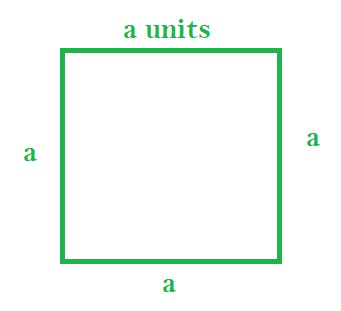# What is the Formula for Perimeter of a Square?

• Last Updated : 20 Dec, 2021

Square is a rectangle whose adjacent sides are equal in length. This means it is a quadrilateral where all the sides are equal in length. Each of the angles in a square is a right angle.

Properties:

• All four sides of a square are equal in length
• The diagonals of a square are equal
• The diagonals of a square bisect each other at right angles
• All the four angles of a square are right angles

Perimeter of a Square

Perimeter refers to the sum of all the sides of a polygon. For a square, it will be the sum of its four equal sides, i.e. four times the length of a side.

Let’s consider a square with each side of length equal to ‘a’ units.

Perimeter = Sum of all sides of square = a + a + a + a = 4a unitsSquare with each side length a units

Thus, the perimeter of a square with the length of each side equal to ‘a’ units is given by 4a.

### Sample Problems

Question 1: Find the perimeter of a square each of side 5 cm.

Solution:

Length of each side of the square = 5 cm

Perimeter of the square = sum of all sides = 5 + 5 + 5 + 5 = 20 cm

Thus, perimeter of square = 20 cm

Question 2: Find the length of sides of a square with a perimeter of 48 cm.

Solution:

Given perimeter of the square = 48 cm

Lets length of each side of the square = ‘a’ cm

Therefore, perimeter = 4a cm

Therefore, we can write:

4a = 48 cm

a = 12 cm

Thus, length of each side of square is 12 cm.

Question 3: Find the perimeter of a square whose length of diagonal is 4√2 cm.

Solution:

Given, the length of a diagonal square = 4√2 cm.

Let the length of each side of the square = a cm.

Thus, applying the Pythagoras theorem, the length of diagonal = √(a2+a2) = a√2 cm

So, we can write:

a√2 = 4√2

a = 4 cm

Thus, the perimeter of square = 4a = 4 × 4 = 16 cm

Question 4: Find the sum of adjacent sides of a square given perimeter of the square is 12 cm.

Solution:

Given the perimeter of the square = 12 cm

Considering length of each side = ‘a’ cm, the perimeter = 4a cm

Thus, we can write:

4a = 12

a = 3 cm

Since adjacent sides of a square are having the same length, we can write the sum of any adjacent sides of the square = a + a = 2a

Therefore, the sum of adjacent sides of the square = 2 × 3 = 6 cm

My Personal Notes arrow_drop_up